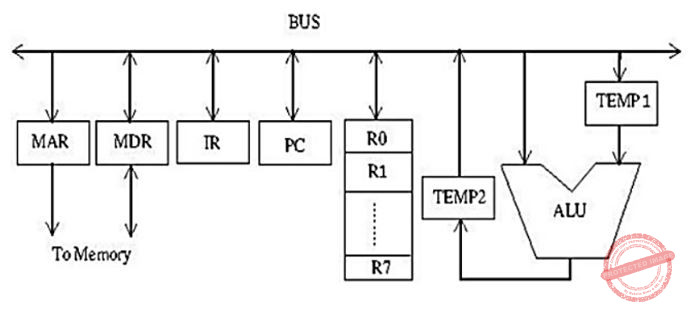October 14, 2023
October 14, 2023
October 14, 2023
###### Computer-Organization
October 14, 2023
 Question 5

Consider the following data path diagram.Consider an instruction: R0 ← R1 + R2. The following steps are used to execute it over the given data path. Assume that PC is incremented appropriately. The subscripts r and w indicate read and write operations, respectively.

```1. R2r, TEMP1r, ALUadd, TEMP2w
2. R1r, TEMP1w
3. PCr, MARw, MEMr
4. TEMP2r, ROw
5. MDRr, IRw ```

Which one of the following is the correct order of execution of the above steps?

 A 3, 5, 1, 2, 4 B 3, 5, 2, 1, 4 C 1, 2, 4, 3, 5 D 2, 1, 4, 5, 3
Question 5 Explanation:
To execute the given instruction R0 ← R1 + R2.

First the PC value has to be moved into MAR (step-3 from the given sequence), then the instruction has to be fetched(step-5 from the given sequence).
Then Temp1 is loaded with the value of R1 (step-2 from the given sequence), then the addition operation is performed by accessing the R2 value directly and adding it to Temp1 value and storing the result in Temp2 (step-1 from the given sequence).
Finally the result from Temp2 is stored in R0 (step-4 from the given sequence).

Hence the correct sequence is (3, 5, 2, 1, 4).

Question 5 Explanation:
To execute the given instruction R0 ← R1 + R2.

First the PC value has to be moved into MAR (step-3 from the given sequence), then the instruction has to be fetched(step-5 from the given sequence).
Then Temp1 is loaded with the value of R1 (step-2 from the given sequence), then the addition operation is performed by accessing the R2 value directly and adding it to Temp1 value and storing the result in Temp2 (step-1 from the given sequence).
Finally the result from Temp2 is stored in R0 (step-4 from the given sequence).

Hence the correct sequence is (3, 5, 2, 1, 4).